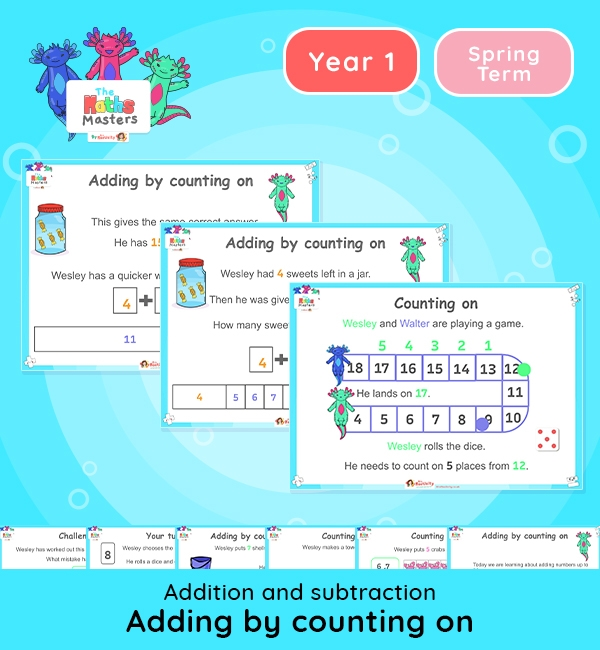# Year 1 | Adding to 20 by Counting on Lesson Presentation## White Rose Year 1 addition and subtraction lesson plans

Aligned with the White Rose Maths scheme of work, this Year 1 | Adding to 20 by Counting on Lesson Presentation is fully editable, and is designed for the Year 1 maths curriculum covering the following maths objectives for the autumn term:

This lesson practises adding by counting on.

It is a longer presentation, so you may wish to split in two. You could allow the children to try some counting on activities using ten frames, cubes, board games, etc before moving on to the second half and working out addition problems by counting on.

White Rose Maths Small Steps: Add by counting on (within 20).

NC Links: * Read, write and interpret mathematical statements involving addition, subtraction and equals signs. *Add and subtract one-digit and two-digit numbers to 20, including zero.

TAF Statements: Working towards: Add and subtract (one digit numbers) explaining their method verbally, in pictures, or using apparatus.

Greater Depth – Use reasoning about number and relationships to solve more complex problems and explain their thinking.

Ready-to-progress criteria:  1NF-1 Develop fluency in addition and subtraction facts within 10

Future applications: Add and subtract across 10.

1AS-2 Read, write and interpret equations containing addition(+), subtraction (-), and equals (=) symbols, and relate additive expressions and equations to real-life contexts.

Previous experience: Devise and record number stories, using pictures, numbers and symbols.

## Recently Viewed